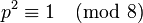# Linear representation theory of direct product of Z8 and Z2

Jump to: navigation, search

## Contents

This article gives specific information, namely, linear representation theory, about a particular group, namely: direct product of Z8 and Z2.
View linear representation theory of particular groups | View other specific information about direct product of Z8 and Z2

## Summary

Item Summary
degrees of irreducible representations over a splitting field 1 (16 times)

maximum: 1, lcm: 1, number: 16, sum of squares: 16
Schur index values of irreducible representations over a splitting field 1,1,1,1,1,1,1,1 (1 occurs 8 times)
Follows from the group being abelian.
smallest ring of realization of irreducible representations (characteristic zero)$\mathbb{Z}[e^{\pi i/4}] = \mathbb{Z}[(1 + i)/\sqrt{2}] = \mathbb{Z}[\cos(\pi/4) + i \sin(\pi/4)]$. Same as$\mathbb{Z}[t]/(t^4 + 1)$.
Same as ring generated by character values
minimal splitting field, i.e., smallest field of realization of irreducible representations (characteristic zero)$\mathbb{Q}(e^{\pi i/4}) = \mathbb{Q}((1 + i)/\sqrt{2}) = \mathbb{Q}(i,\sqrt{2})$. Same as$\mathbb{Q}[t]/(t^4 + 1)$.
Same as field generated by character values, also the unique smallest sufficiently large field. All coincide because the group is abelian.
condition for a field to be a splitting field Characteristic not$2$, and the polynomial$t^4 + 1$ should split over the field.
For a finite field of size$q$, equivalent to$8$ dividing$q - 1$
minimal splitting field in characteristic$p \ne 0,2$ Case$p \equiv 1 \pmod 8$: prime field$\mathbb{F}_p$
Case$p \equiv 3,5,7 \pmod 8$:$\mathbb{F}_{p^2}$, quadratic extension of$\mathbb{F}_p$. This is because$p^2 \equiv 1 \pmod 8$ in all cases.
smallest size splitting field field:F9
degrees of irreducible representations over a non-splitting field, such as the field of real numbers$\R$, the field of rational numbers$\mathbb{Q}$  ?
orbits of irreducible representations over a splitting field under the action of the automorphism group  ?